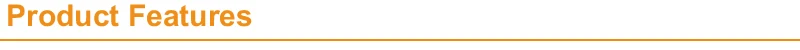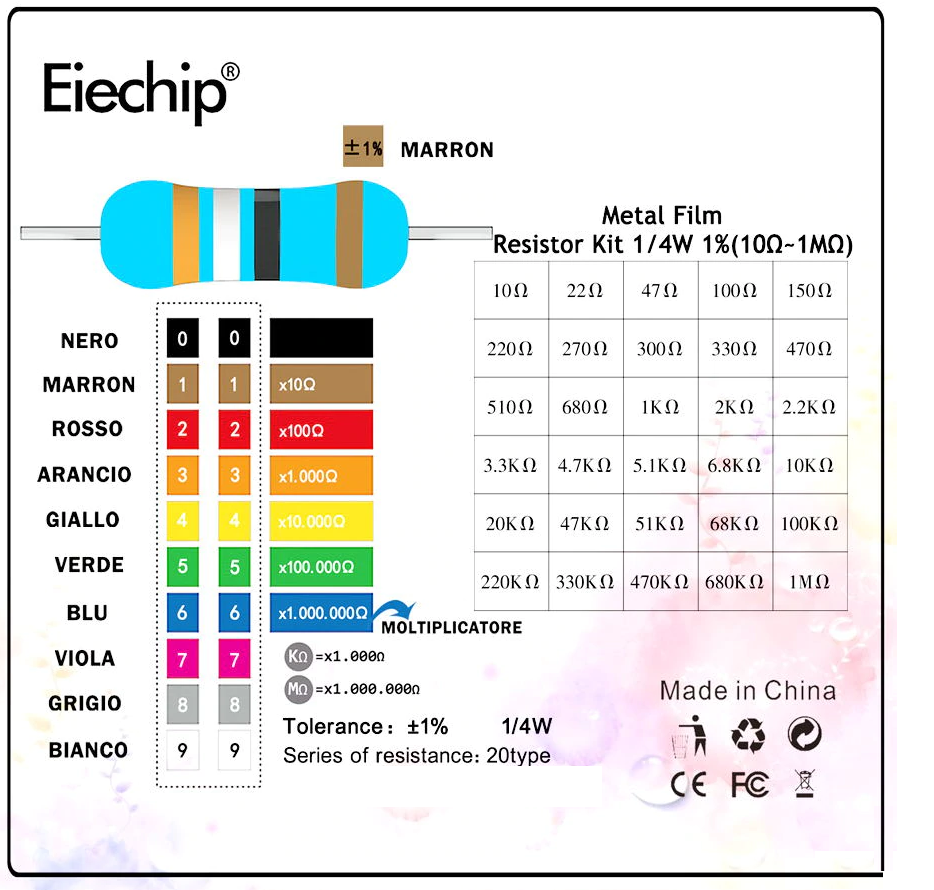# 1R-1201MR 1ohm-120Mohm Resistor

(No reviews yet)
SKU:
BEMFR55
Weight:
0.03 KGS
Shipping:
Calculated at Checkout
\$0.02

## DescriptionSpecification:
Color:As Pictures
Structure:Fixed capacitors
Kinds:Aluminum Electrolytic Capacitors

Features:
Durable and easy to install
Very convenient and practical
Very popular among the electronic lovers0.1 ohm    0.12 ohm    0.13 ohm    0.15 ohm    0.16 ohm    0.18 ohm    0.2 ohm    0.22 ohm    0.24 ohm   8.2 ohm   9.1 ohm    10 ohm   11 ohm   12 ohm   1.3M ohm    1.5M ohm   1.6M ohm   1.8M ohm    2M ohm   2.2M ohm   2.4M ohm   2.7M ohm    3M ohm   3.3M ohm    3.6M ohm    3.9M ohm    4.3M ohm   4.7M ohm   5.1M ohm    5.6M ohm   6.2M ohm   6.8M ohm   91K ohm 0.27 ohm    0.3 ohm   0.33 ohm   0.36 ohm    0.39 ohm    0.43 ohm    0.47 ohm    0.5 ohm   0.56 ohm   13 ohm    15 ohm   16 ohm   18 ohm   20 ohm   910K ohm   1M ohm   1.1M ohm   1.2M ohm   10M ohm    12M ohm   15M ohm    18M ohm    20M ohm    7.5M ohm    8.2M ohm    9.1M ohm   47K ohm    51K ohm   56K ohm   62K ohm    68K ohm   75K ohm   82K ohm 0.62 ohm   0.68 ohm   0.75 ohm   0.82 ohm   0.91 ohm    1 ohm    1.1 ohm    1.2 ohm    1.3 ohm   22 ohm   24 ohm    27 ohm   30 ohm    33 ohm   8.2K ohm   9.1K ohm   10K ohm    11K ohm   12K ohm   13K ohm    15K ohm   16K ohm   18K ohm   20K ohm   22K ohm    24K ohm    27K ohm    30K ohm    33K ohm   36K ohm    39K ohm   43K ohm   7.5K ohm 1.5 ohm    1.6 ohm   1.8 ohm   2 ohm    2.2 ohm    2.4 ohm   2.7 ohm   3 ohm   3.3 ohm   36 ohm   39 ohm   43 ohm    47 ohm   51 ohm   56 ohm    62 ohm   68 ohm   75 ohm   82 ohm   91 ohm   100 ohm   100K ohm   110K ohm    120K ohm   130K ohm   150K ohm   160K ohm   180K ohm    200K ohm    220K ohm   240K ohm    270K ohm   6.8K ohm 3.6 ohm   3.9 ohm    4.3 ohm   4.7 ohm    5.1 ohm   5.6 ohm    6.2 ohm    6.8 ohm   7.5 ohm   110 ohm    120 ohm   130 ohm   150 ohm   160 ohm   180 ohm   200 ohm   220 ohm   240 ohm   270 ohm    300 ohm   300K ohm    330K ohm    360K ohm   390K ohm   430K ohm   470K ohm    510K ohm    560K ohm   620K ohm   680K ohm   750K ohm    820K ohm 330 ohm   360 ohm    390 ohm   430 ohm   470 ohm    510 ohm   560 ohm   620 ohm   680 ohm   750 ohm   820 ohm   910 ohm   1K ohm    1.1K ohm   1.2K ohm   1.3K ohm   1.5K ohm   1.6K ohm    1.8K ohm   2K ohm   2.2K ohm    2.4K ohm   2.7K ohm   3K ohm    3.3K ohm   3.6K ohm   3.9K ohm   4.3K ohm    4.7K ohm    5.1K ohm   5.6K ohm    6.2K ohm
View AllClose

View AllClose